/

### Showing that the Mean Excess Function of a Generalised Pareto Distribution is linear in the exceedance threshold (for a specific range of values of the distribution’s shape parameter)

If a random variable,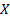, is distributed according to a generalised Pareto distribution,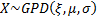, then it has the following probability density function (for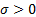):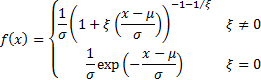If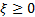then its domain is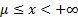.

The mean excess function of a probability distribution is defined as: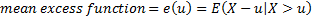If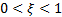then then mean excess function for this distribution is as follows (for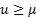):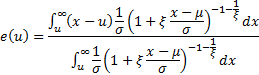Let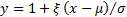so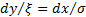and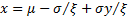. Let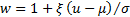. Then: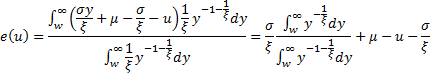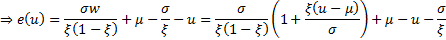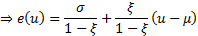This is linear in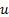as desired. A consequence is that we can test visually whether a data set appears to be coming from a GPD by plotting the empirical mean excess function and seeing if it appears to be linear (and we can also estimate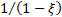from its slope if it is linear).